# Deep Network Quantizer

Quantize a deep neural network to 8-bit scaled integer data types

Since R2020a

## Description

Use the Deep Network Quantizer app to reduce the memory requirement of a deep neural network by quantizing weights, biases, and activations of layers to 8-bit scaled integer data types. Using this app you can:

• Visualize the dynamic ranges of convolution layers in a deep neural network.

• Select individual network layers to quantize.

• Assess the performance of a quantized network.

• Generate GPU code to deploy the quantized network using GPU Coder™.

• Generate HDL code to deploy the quantized network to an FPGA using Deep Learning HDL Toolbox™.

• Generate C++ code to deploy the quantized network to an ARM Cortex-A microcontroller using MATLAB® Coder™.

• Generate a simulatable quantized network that you can explore in MATLAB without generating code or deploying to hardware.

This app requires Deep Learning Toolbox Model Quantization Library. To learn about the products required to quantize a deep neural network, see Quantization Workflow Prerequisites.

## Open the Deep Network Quantizer App

• MATLAB command prompt: Enter `deepNetworkQuantizer`.

• MATLAB toolstrip: On the Apps tab, under Machine Learning and Deep Learning, click the app icon.

## Examples

expand all

To explore the behavior of a neural network with quantized convolution layers, use the Deep Network Quantizer app. This example quantizes the learnable parameters of the convolution layers of the `squeezenet` neural network after retraining the network to classify new images according to the Train Deep Learning Network to Classify New Images example.

This example uses a DAG network with the GPU execution environment.

Load the network to quantize into the base workspace.

```load squeezenetmerch net```
```net = DAGNetwork with properties: Layers: [68×1 nnet.cnn.layer.Layer] Connections: [75×2 table] InputNames: {'data'} OutputNames: {'new_classoutput'} ```

Define calibration and validation data.

The app uses calibration data to exercise the network and collect the dynamic ranges of the weights and biases in the convolution and fully connected layers of the network and the dynamic ranges of the activations in all layers of the network. For the best quantization results, the calibration data must be representative of inputs to the network.

The app uses the validation data to test the network after quantization to understand the effects of the limited range and precision of the quantized learnable parameters of the convolution layers in the network.

In this example, use the images in the `MerchData` data set. Define an `augmentedImageDatastore` object to resize the data for the network. Then, split the data into calibration and validation data sets.

```unzip('MerchData.zip'); imds = imageDatastore('MerchData', ... 'IncludeSubfolders',true, ... 'LabelSource','foldernames'); [calData, valData] = splitEachLabel(imds, 0.7, 'randomized'); aug_calData = augmentedImageDatastore([227 227], calData); aug_valData = augmentedImageDatastore([227 227], valData);```

At the MATLAB command prompt, open the app.

```deepNetworkQuantizer ```

In the app, click New and select `Quantize a network`.

In the dialog, select the execution environment and the network to quantize from the base workspace. For this example, select a GPU execution environment and the DAG network, `net`.The app displays the layer graph of the selected network.

In the Calibrate section of the toolstrip, under Calibration Data, select the `augmentedImageDatastore` object from the base workspace containing the calibration data, `aug_calData`. Select Calibrate.

The Deep Network Quantizer uses the calibration data to exercise the network and collect range information for the learnable parameters in the network layers.

When the calibration is complete, the app displays a table containing the weights and biases in the convolution and fully connected layers of the network and the dynamic ranges of the activations in all layers of the network and their minimum and maximum values during the calibration. To the right of the table, the app displays histograms of the dynamic ranges of the parameters. The gray regions of the histograms indicate data that cannot be represented by the quantized representation. For more information on how to interpret these histograms, see Quantization of Deep Neural Networks.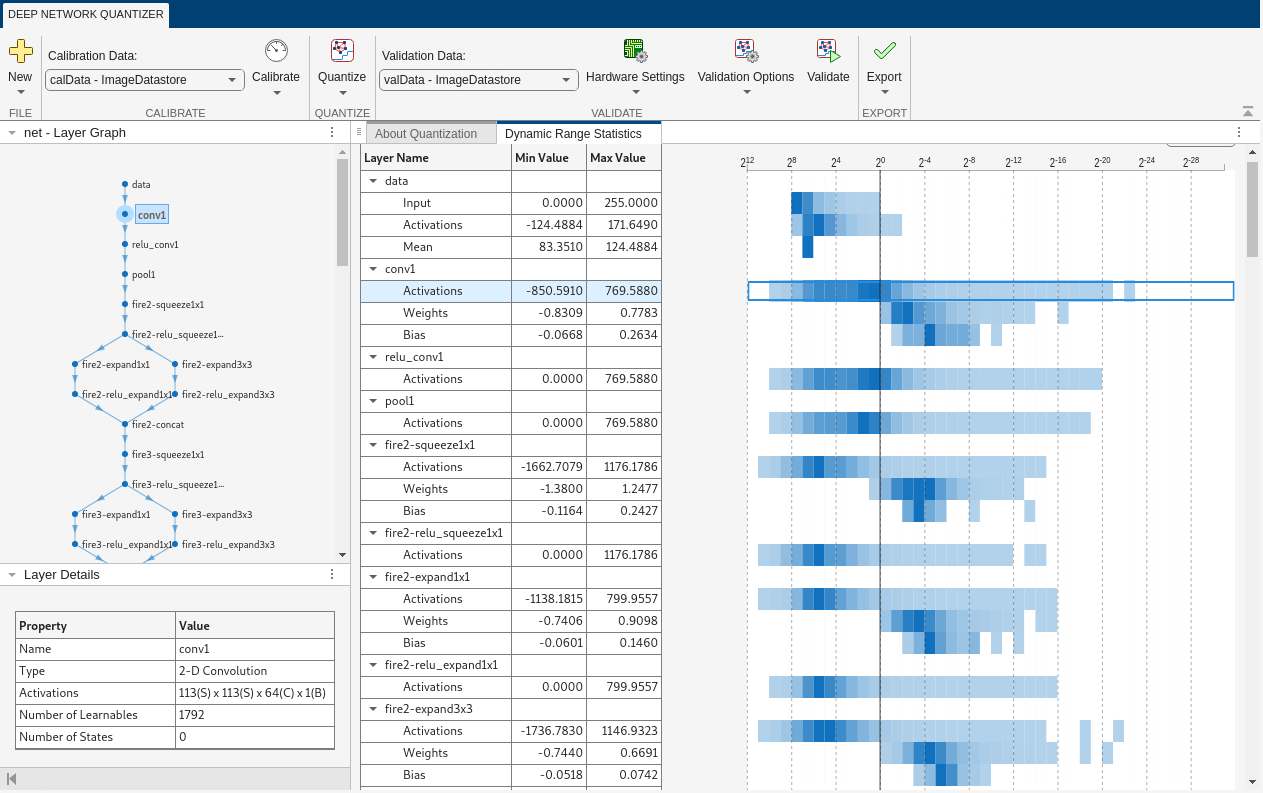In the Quantize column of the table, indicate whether to quantize the learnable parameters in the layer. Layers that are not quantized remain in single-precision after quantization.

In the Validate section of the toolstrip, under Validation Data, select the `augmentedImageDatastore` object from the base workspace containing the validation data, `aug_valData`.

In the Validate section of the toolstrip, under Quantization Options, select the Default metric function and MinMax exponent scheme. Select Quantize and Validate.

The Deep Network Quantizer quantizes the weights, activations, and biases of convolution layers in the network to scaled 8-bit integer data types and uses the validation data to exercise the network. The app determines a default metric function to use for the validation based on the type of network that is being quantized. For a classification network, the app uses Top-1 Accuracy.

When the validation is complete, the app displays the results of the validation, including:

• Metric function used for validation

• Result of the metric function before and after quantization

• Memory requirement of the network before and after quantization (MB)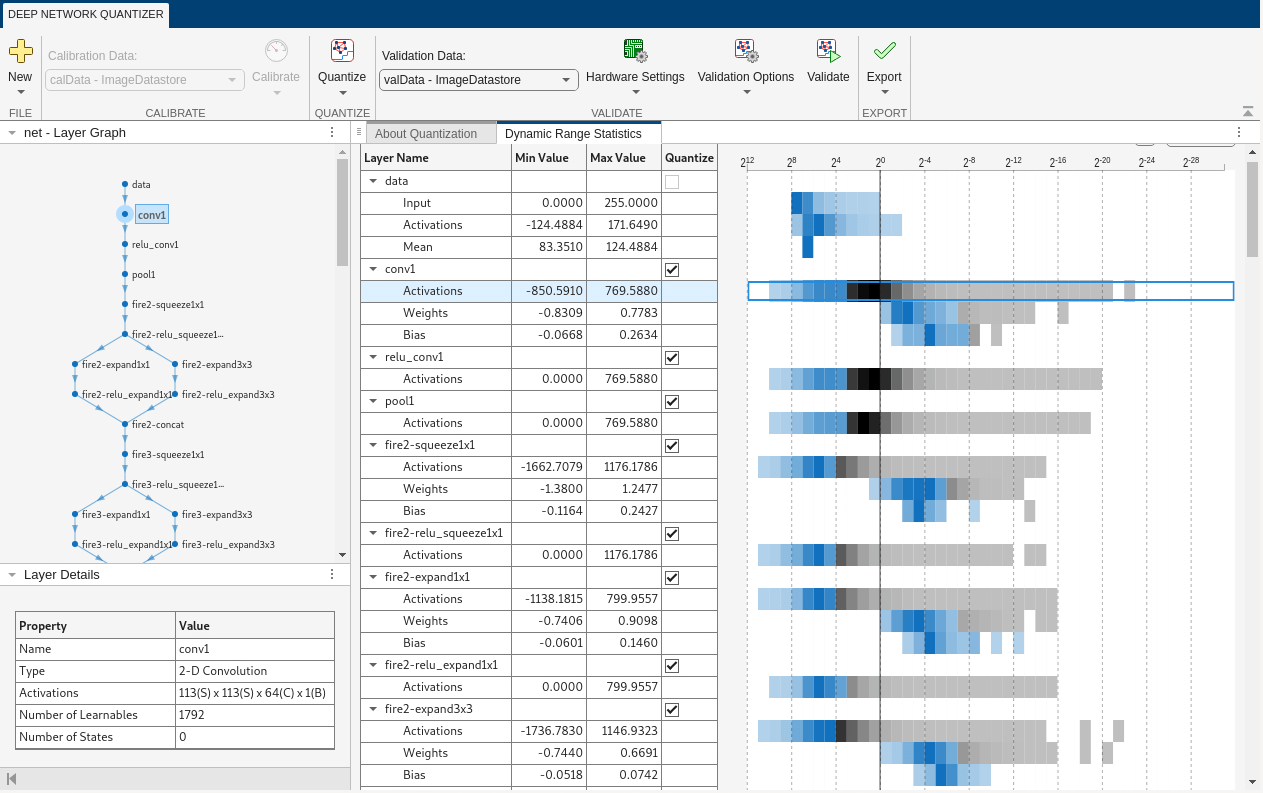If you want to use a different metric function for validation, for example to use the Top-5 accuracy metric function instead of the default Top-1 accuracy metric function, you can define a custom metric function. Save this function in a local file.

```function accuracy = hComputeModelAccuracy(predictionScores, net, dataStore) %% Computes model-level accuracy statistics % Load ground truth tmp = readall(dataStore); groundTruth = tmp.response; % Compare with predicted label with actual ground truth predictionError = {}; for idx=1:numel(groundTruth) [~, idy] = max(predictionScores(idx,:)); yActual = net.Layers(end).Classes(idy); predictionError{end+1} = (yActual == groundTruth(idx)); %#ok end % Sum all prediction errors. predictionError = [predictionError{:}]; accuracy = sum(predictionError)/numel(predictionError); end ```

To revalidate the network using this custom metric function, under Quantization Options, enter the name of the custom metric function, `hComputeModelAccuracy`. Select Add to add `hComputeModelAccuracy` to the list of metric functions available in the app. Select `hComputeModelAccuracy` as the metric function to use.

The custom metric function must be on the path. If the metric function is not on the path, this step will produce an error.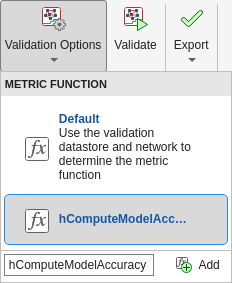Select Quantize and Validate.

The app quantizes the network and displays the validation results for the custom metric function.The app displays only scalar values in the validation results table. To view the validation results for a custom metric function with non-scalar output, export the `dlquantizer` object as described below, then validate using the `validate` function at the MATLAB command window.

If the performance of the quantized network is not satisfactory, you can choose to not quantize some layers by deselecting the layer in the table. You can also explore the effects of choosing a different exponent selection scheme for quantization in the Quantization Options menu. To see the effects of these changes, select Quantize and Validate again.

After calibrating the network, you can choose to export the quantized network or the `dlquantizer` object. Select the Export button. In the drop down, select from the following options:

• Export Quantized Network - Add the quantized network to the base workspace. This option exports a simulatable quantized network that you can explore in MATLAB without deploying to hardware.

• Export Quantizer - Add the `dlquantizer` object to the base workspace. You can save the `dlquantizer` object and use it for further exploration in the Deep Network Quantizer app or at the command line, or use it to generate code for your target hardware.

• Generate Code - Open the GPU Coder app and generate GPU code from the quantized neural network. Generating GPU code requires a GPU Coder™ license.

To explore the behavior of a neural network with quantized convolution layers, use the Deep Network Quantizer app. This example quantizes the learnable parameters of the convolution layers of the `squeezenet` neural network after retraining the network to classify new images according to the Train Deep Learning Network to Classify New Images example.

This example uses a DAG network with the CPU execution environment.

Load the network to quantize into the base workspace.

```load squeezenetmerch net```
```net = DAGNetwork with properties: Layers: [68×1 nnet.cnn.layer.Layer] Connections: [75×2 table] InputNames: {'data'} OutputNames: {'new_classoutput'} ```

Define calibration and validation data.

The app uses calibration data to exercise the network and collect the dynamic ranges of the weights and biases in the convolution and fully connected layers of the network and the dynamic ranges of the activations in all layers of the network. For the best quantization results, the calibration data must be representative of inputs to the network.

The app uses the validation data to test the network after quantization to understand the effects of the limited range and precision of the quantized learnable parameters of the convolution layers in the network.

In this example, use the images in the `MerchData` data set. Define an `augmentedImageDatastore` object to resize the data for the network. Then, split the data into calibration and validation data sets.

```unzip('MerchData.zip'); imds = imageDatastore('MerchData', ... 'IncludeSubfolders',true, ... 'LabelSource','foldernames'); [calData, valData] = splitEachLabel(imds, 0.7, 'randomized'); aug_calData = augmentedImageDatastore([227 227], calData); aug_valData = augmentedImageDatastore([227 227], valData);```

At the MATLAB command prompt, open the app.

```deepNetworkQuantizer ```

In the app, click New and select `Quantize a network`.

In the dialog, select the execution environment and the network to quantize from the base workspace. For this example, select a CPU execution environment and the DAG network, `net`.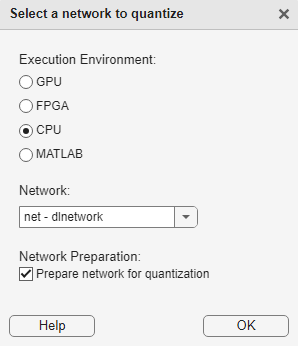The app displays the layer graph of the selected network.

In the Calibrate section of the toolstrip, under Calibration Data, select the `augmentedImageDatastore` object from the base workspace containing the calibration data, `aug_calData`. Select Calibrate.

The Deep Network Quantizer uses the calibration data to exercise the network and collect range information for the learnable parameters in the network layers.

When the calibration is complete, the app displays a table containing the weights and biases in the convolution and fully connected layers of the network and the dynamic ranges of the activations in all layers of the network and their minimum and maximum values during the calibration. To the right of the table, the app displays histograms of the dynamic ranges of the parameters. The gray regions of the histograms indicate data that cannot be represented by the quantized representation. For more information on how to interpret these histograms, see Quantization of Deep Neural Networks.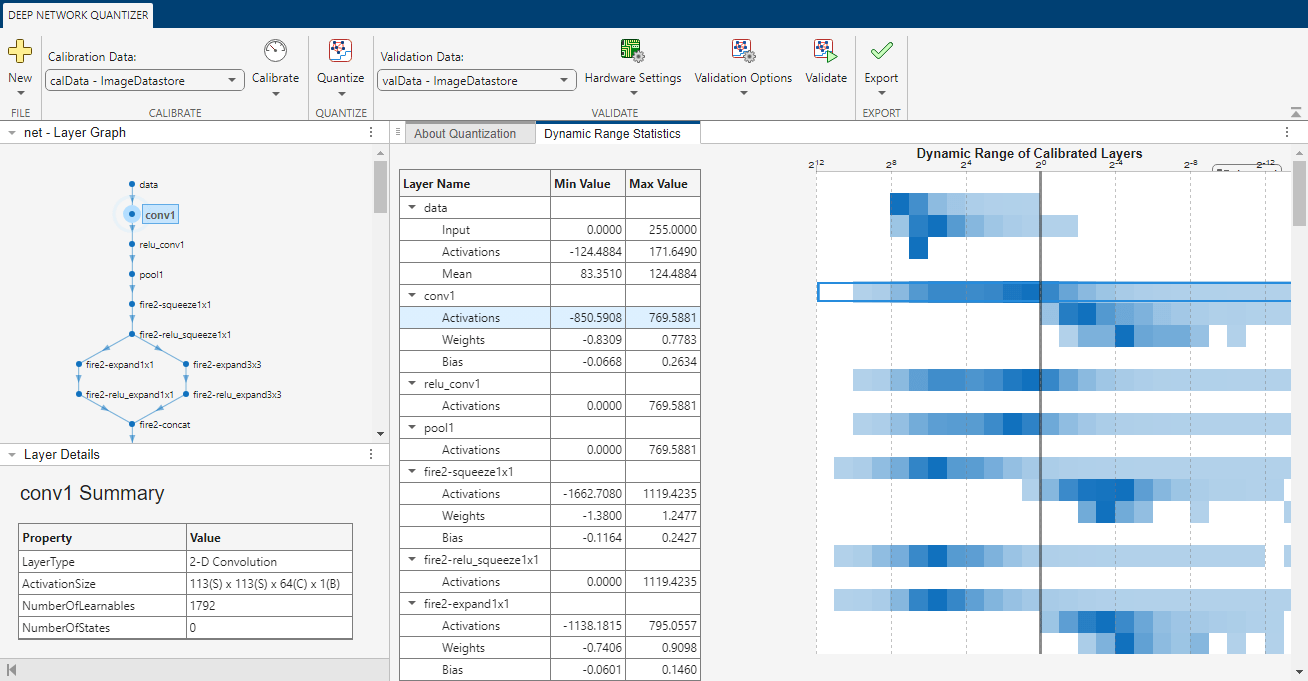In the Quantize column of the table, indicate whether to quantize the learnable parameters in the layer. Layers that are not quantized remain in single-precision after quantization.

In the Validate section of the toolstrip, under Validation Data, select the `augmentedImageDatastore` object from the base workspace containing the validation data, `aug_valData`.

In the Validate section of the toolstrip, under Hardware Settings, select Raspberry Pi as the Simulation Environment. The app auto-populates the Target credentials from an existing connection or from the last successful connection. You can also use this option to create a new Raspberry Pi connection.In the Validate section of the toolstrip, under Quantization Options, select the Default metric function and MinMax exponent scheme. Select Quantize and Validate.

The Deep Network Quantizer quantizes the weights, activations, and biases of convolution layers in the network to scaled 8-bit integer data types and uses the validation data to exercise the network. The app determines a default metric function to use for the validation based on the type of network that is being quantized. For a classification network, the app uses Top-1 Accuracy.

When the validation is complete, the app displays the results of the validation, including:

• Metric function used for validation

• Result of the metric function before and after quantization

• Memory requirement of the network before and after quantization (MB)If the performance of the quantized network is not satisfactory, you can choose to not quantize some layers by deselecting the layer in the table. You can also explore the effects of choosing a different exponent selection scheme for quantization in the Quantization Options menu. To see the effects of these changes, select Quantize and Validate again.

After calibrating the network, you can choose to export the quantized network or the `dlquantizer` object. Select the Export button. In the drop down, select from the following options:

• Export Quantized Network - Add the quantized network to the base workspace. This option exports a simulatable quantized network that you can explore in MATLAB without deploying to hardware.

• Export Quantizer - Add the `dlquantizer` object to the base workspace. You can save the `dlquantizer` object and use it for further exploration in the Deep Network Quantizer app or at the command line, or use it to generate code for your target hardware.

• Generate Code - Open the MATLAB Coder app and generate C++ code from the quantized neural network. Generating C++ code requires a MATLAB Coder™ license.

To explore the behavior of a neural network that has quantized convolution layers, use the Deep Network Quantizer app. This example quantizes the learnable parameters of the convolution layers of the `LogoNet` neural network for an FPGA target.

For this example, you need the products listed under `FPGA` in Quantization Workflow Prerequisites.

Load the pretrained network to quantize into the base workspace. Create a file in your current working folder called `getLogoNetwork.m`. In the file, enter:

```function net = getLogoNetwork if ~isfile('LogoNet.mat') url = 'https://www.mathworks.com/supportfiles/gpucoder/cnn_models/logo_detection/LogoNet.mat'; websave('LogoNet.mat',url); end data = load('LogoNet.mat'); net = data.convnet; end ```

`snet = getLogoNetwork;`
```snet = SeriesNetwork with properties: Layers: [22×1 nnet.cnn.layer.Layer] InputNames: {'imageinput'} OutputNames: {'classoutput'}```

Define calibration and validation data to use for quantization.

The Deep Network Quantizer app uses calibration data to exercise the network and collect the dynamic ranges of the weights and biases in the convolution and fully connected layers of the network. The app also exercises the dynamic ranges of the activations in all layers of the LogoNet network. For the best quantization results, the calibration data must be representative of inputs to the LogoNet network.

After quantization, the app uses the validation data set to test the network to understand the effects of the limited range and precision of the quantized learnable parameters of the convolution layers in the network.

In this example, use the images in the `logos_dataset` data set to calibrate and validate the LogoNet network. Define an `imageDatastore` object, then split the data into calibration and validation data sets.

Expedite the calibration and validation process for this example by using a subset of the calibration and validation data. Store the new reduced calibration data set in `calData_concise` and the new reduced validation data set in `valData_concise`.

```currentDir = pwd; openExample('deeplearning_shared/QuantizeNetworkForFPGADeploymentExample') unzip('logos_dataset.zip'); imds = imageDatastore(fullfile(currentDir,'logos_dataset'),... 'IncludeSubfolders',true,'FileExtensions','.JPG','LabelSource','foldernames'); [calData,valData] = splitEachLabel(imds,0.7,'randomized'); calData_concise = calData.subset(1:20); valData_concise = valData.subset(1:6);```

Open the Deep Network Quantizer app.

`deepNetworkQuantizer`

Click and select ```Quantize a network```.

Set the execution environment to FPGA and select ```snet - SeriesNetwork``` as the network to quantize.The app displays the layer graph of the selected network.

Under Calibration Data, select the ```calData_concise - ImageDatastore``` object from the base workspace containing the calibration data.

Click . By default, the app uses the host GPU to collect calibration data, if one is available. Otherwise, the host CPU is used. You can use the drop down menu to select the calibration environment.

The Deep Network Quantizer app uses the calibration data to exercise the network and collect range information for the learnable parameters in the network layers.

When the calibration is complete, the app displays a table containing the weights and biases in the convolution and fully connected layers of the network. Also displayed are the dynamic ranges of the activations in all layers of the network and their minimum and maximum values recorded during the calibration. The app displays histograms of the dynamic ranges of the parameters. The gray regions of the histograms indicate data that cannot be represented by the quantized representation. For more information on how to interpret these histograms, see Quantization of Deep Neural Networks.In the Quantize Layer column of the table, indicate whether to quantize the learnable parameters in the layer. Layers that are not quantized remain in single-precision.

Under Validation Data, select the ```valData_concise - ImageDatastore``` object from the base workspace containing the validation data.

In the Hardware Settings section of the toolstrip, select the environment to use for validation of the quantized network. For more information on these options, see Hardware Settings.

For this example, select Xilinx ZC706 (zc706_int8) and JTAG.Under , select the Default metric function and MinMax exponent scheme. For more information on these options, see Quantization Options.

Click .

The Deep Network Quantizer app quantizes the weights, activations, and biases of convolution layers in the network to scaled 8-bit integer data types and uses the validation data to exercise the network. The app determines a default metric function to use for the validation based on the type of network that is being quantized. For more information, see Quantization Options.

When the validation is complete, the app displays the validation results.After quantizing and validating the network, you can choose to export the quantized network.

Click the button. In the drop-down list, select Export Quantizer to create a `dlquantizer` object in the base workspace. You can deploy the quantized network to your target FPGA board and retrieve the prediction results by using MATLAB. For an example, see Classify Images on FPGA Using Quantized Neural Network (Deep Learning HDL Toolbox).

This example shows you how to import a `dlquantizer` object from the base workspace into the Deep Network Quantizer app. This allows you to begin quantization of a deep neural network using the command line or the app, and resume your work later in the app.

Open the Deep Network Quantizer app.

`deepNetworkQuantizer`

In the app, click and select `Import dlquantizer object`.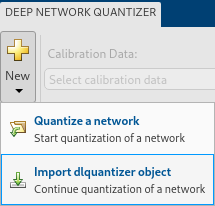In the dialog, select the `dlquantizer` object to import from the base workspace. For this example, use `quantObj` that you create in the above example Quantize a Neural Network for GPU Target.The app imports any data contained in the `dlquantizer` object that was collected at the command line. This data can include the network to quantize, calibration data, validation data, and calibration statistics.

The app displays a table containing the calibration data contained in the imported `dlquantizer` object, `quantObj`. To the right of the table, the app displays histograms of the dynamic ranges of the parameters. The gray regions of the histograms indicate data that cannot be represented by the quantized representation. For more information on how to interpret these histograms, see Quantization of Deep Neural Networks.## Parameters

expand all

When you select New > Quantize a Network, the app allows you to choose the execution environment for the quantized network. How the network is quantized depends on the choice of execution environment.

When you select the `MATLAB` execution environment, the app performs target-agnostic quantization of the neural network. This option does not require you to have target hardware in order to explore the quantized network in MATLAB.

Specify hardware settings based on your execution environment.

• GPU Execution Environment

Select from the following simulation environments:

Simulation EnvironmentAction

GPU

Simulate on host GPU

Deploys the quantized network to the host GPU. Validates the quantized network by comparing performance to single-precision version of the network.

MATLAB

Simulate in MATLAB

Simulates the quantized network in MATLAB. Validates the quantized network by comparing performance to single-precision version of the network.

• FPGA Execution Environment

Select from the following simulation environments:

Simulation EnvironmentAction

Cosimulation

Emulate on host

Emulates the quantized network in MATLAB. Validates the quantized network by comparing performance to single-precision version of the network.

Intel Arria 10 SoC

arria10soc_int8

Deploys the quantized network to an Intel® Arria® 10 SoC board by using the `arria10soc_int8` bitstream. Validates the quantized network by comparing performance to single-precision version of the network.

Xilinx ZCU102

zcu102_int8

Deploys the quantized network to a Xilinx® Zynq® UltraScale+™ MPSoC ZCU102 10 SoC board by using the `zcu102_int8` bitstream. Validates the quantized network by comparing performance to single-precision version of the network.

Xilinx ZC706

zc706_int8

Deploys the quantized network to a Xilinx Zynq-7000 ZC706 board by using the `zc706_int8` bitstream. Validates the quantized network by comparing performance to single-precision version of the network.

When you select the Intel Arria 10 SoC, Xilinx ZCU102, or Xilinx ZC706 option, additionally select the interface to use to deploy and validate the quantized network.

Target OptionAction
JTAGPrograms the target FPGA board selected under Simulation Environment by using a JTAG cable. For more information, see JTAG Connection (Deep Learning HDL Toolbox).
EthernetPrograms the target FPGA board selected in Simulation Environment through the Ethernet interface. Specify the IP address for your target board in the IP Address field.

• CPU Execution Environment

Select from the following simulation environments:

Simulation EnvironmentAction

Raspberry Pi

Deploys the quantized network to the Raspberry Pi board. Validates the quantized network by comparing performance to single-precision version of the network.

When you select the Raspberry Pi option, additionally specify the following details for the `raspi` connection.

Target OptionDescription
HostnameHostname of the board, specified as a string.

By default, the Deep Network Quantizer app determines a metric function to use for the validation based on the type of network that is being quantized.

Type of NetworkMetric Function
Classification

Top-1 Accuracy – Accuracy of the network

Object Detection

Average Precision – Average precision over all detection results. See `evaluateDetectionPrecision` (Computer Vision Toolbox).

Regression

MSE – Mean squared error of the network

Semantic Segmentation

WeightedIOU – Average IoU of each class, weighted by the number of pixels in that class. See `evaluateSemanticSegmentation` (Computer Vision Toolbox).

You can also specify a custom metric function to use for validation.

You can select the exponent selection scheme to use for quantization of the network:

• MinMax — (default) Evaluate the exponent based on the range information in the calibration statistics and avoid overflows.

• Histogram — Distribution-based scaling which evaluates the exponent to best fit the calibration data.

• Export Quantized Network — After calibrating the network, quantize and add the quantized network to the base workspace. This option exports a simulatable quantized network, `quantizedNet`, that you can explore in MATLAB without deploying to hardware. This option is equivalent to using `quantize` at the command line.

Code generation is not supported for the exported quantized network, `quantizedNet`.

• Export Quantizer — Add the `dlquantizer` object to the base workspace. You can save the `dlquantizer` object and use it for further exploration in the Deep Network Quantizer app or at the command line, or use it to generate code for your target hardware.

• Generate Code

Execution EnvironmentCode Generation
GPUOpen the GPU Coder app and generate GPU code from the quantized and validated neural network. Generating GPU code requires a GPU Coder license.
CPUOpen the MATLAB Coder app and generate C++ code from the quantized and validated neural network. Generating C++ code requires a MATLAB Coder license.

## Limitations

• Validation on target hardware for CPU, FPGA, and GPU execution environments is not supported in MATLAB Online™. For FPGA and GPU execution environments, validation can be performed through emulation on the MATLAB Online host. GPU validation can also be performed if GPU support has been added to your MATLAB Online Server™ cluster. For more information on GPU support for MATLAB Online, see Configure GPU Support in MATLAB Online Server (MATLAB Online Server).

## Version History

Introduced in R2020a

expand all